## How to sumif with one or more criteria in Excel?

In Excel, sum values based on one or more criteria is a common task for most of us, the SUMIF function can help us to quickly sum the values based on one condition and the SUMIFS function help us to sum values with multiple criteria. This article, I will describe how to sum with one or more criteria in Excel?

Sum cell values with one criterion in Excel

Sum cell values with multiple criteria in Excel

####Sum cell values with one criterion in Excel

First, I will give the SUMIF syntax to you for reference:

SUMIF(range, criteria, [sum_range])

• Range: the range of cells to be evaluated by your criteria;
• Criteria: the condition that you want to use;
• Sum_range: the range of cells to sum if the condition is met

For applying this SUMIF function, I will take the following data for example: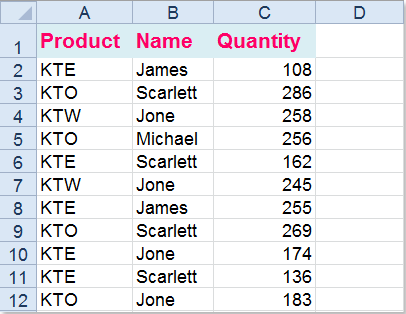(1.) Sum of quantity when the product is “KTE”:

=SUMIF(A2:A12,E2,C2:C12) and press Enter key to get the result, (A2:A12 is the range cells which contains the criteria, E2 is the criterion you want to sum the values based on, C2:C12 refers to the range you want to sum) see screenshot: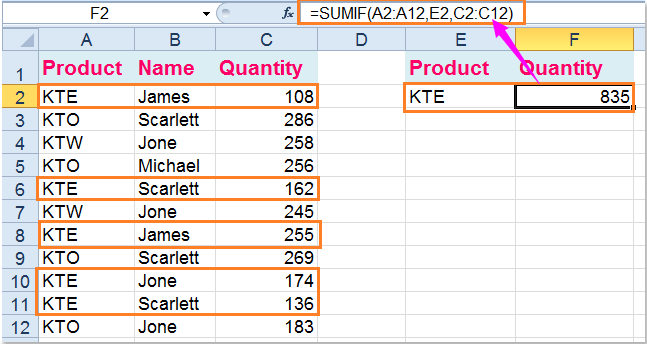=SUMIF(A2:A12,"<>KTE",C2:C12), then press Enter key to add up all product quantity excluding KTE, (A2:A12 is the range cells which contains the criteria, <>KTE is the criterion which excludes KTE, C2:C12 refers to the range you want to sum), see screenshot: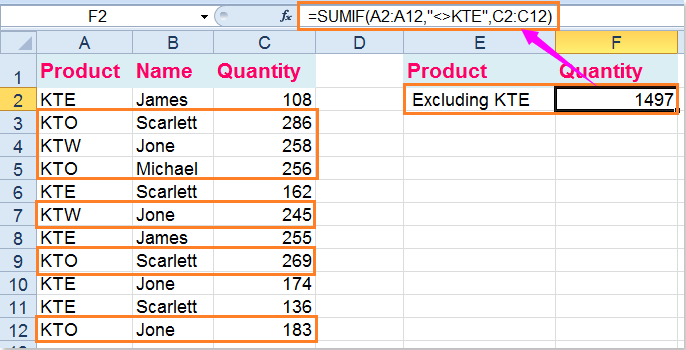(3.) Sum the quantity greater than 250:

=SUMIF(C2:C12,">250"), and press Enter key to get the result, (C2:C12 refers to the range you want to sum, >250 is the criterion you need, you also can change it to less than 250 as you need), see screenshot: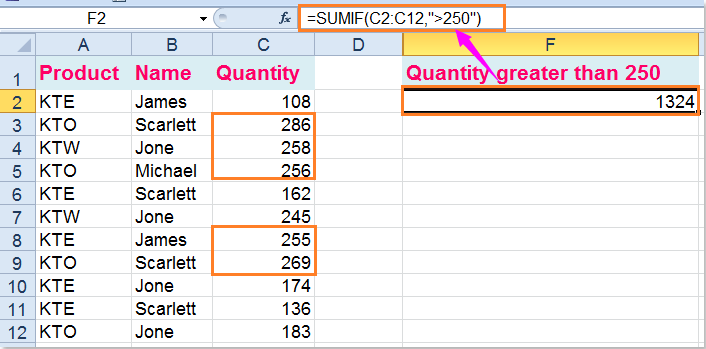(4.) Use SUMIF+SUMIF+… function with multiple OR criteria, sum the quantity of KTE and KTW:

=SUMIF(A2:A12,"KTE",C2:C12) + SUMIF(A2:A12,"KTW",C2:C12)( A2:A12 is the range cells which contains the criteria, KTE and KTW are the criteria you want to sum the values based on, C2:C12 refers to the range you want to sum), and then press Enter key to get the result: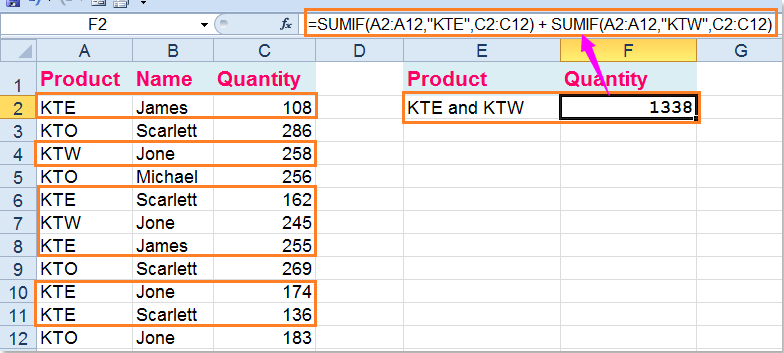####Sum cell values with multiple criteria in Excel

The SUMIFS syntax as follows:

SUMIFS(sum_range, criteria_range1, criteria1,[criteria_range2, criteria2],...)

• Sum_range: a range of cells to sum;
• Criteria_range1: the first range which has the criteria;
• criteria1: the first condition that must be met;
• criteria_range2, criteria2, ...: these are additional ranges and criteria associated with them.

(1.) Sum the quantity where the product is KTE and name is James:

Please enter this formula: =SUMIFS(C2:C12,A2:A12,"KTE",B2:B12,"James"),(C2:C12 is the range of cells need to sum, A2:A12 , KTE are the first criteria range and criterion, B2:B12, James, are the second criteria range and criterion), and then press Enter key, see screenshot: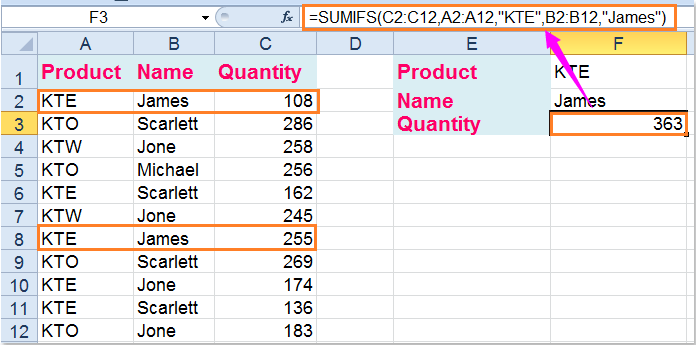Here also has an array formula can help you: =SUM((A2:A12="kte")*(B2:B12="James")*C2:C12), and you need to press Ctrl + Shift + Enter keys together after typing the formula.

(2.) Sum the quantity where the product is KTE and name is not James

Type this formula: =SUMIFS(C2:C12,A2:A12,"KTE",B2:B12,"<>James")(C2:C12 is the range of cells need to sum, A2:A12 , KTE are the first criteria range and criterion, B2:B12, <>James, are the second criteria range and criterion), then press Enter key to get the result, see screenshot: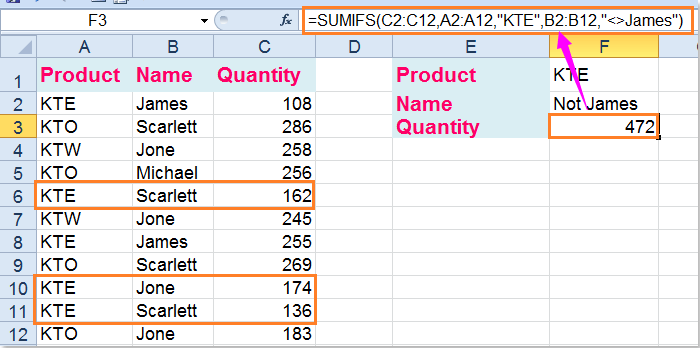Another array formula also can finish this operation: =SUM((A2:A12="kte")*(B2:B12<>"James")*C2:C12), but you must press Ctrl + Shift + Enter keys after typing this formula.

(3.) Sum the quantity where the product is KTE and quantity greater than 150

You can use this SUMIFS formula: =SUMIFS(C2:C12,A2:A12,"KTE",C2:C12,">150")(C2:C12 is the range of cells need to sum, A2:A12 , KTE are the first criteria range and criterionj, C2:C12, >150, are the second criteria range and criterion), and then tap the Enter key to return the result.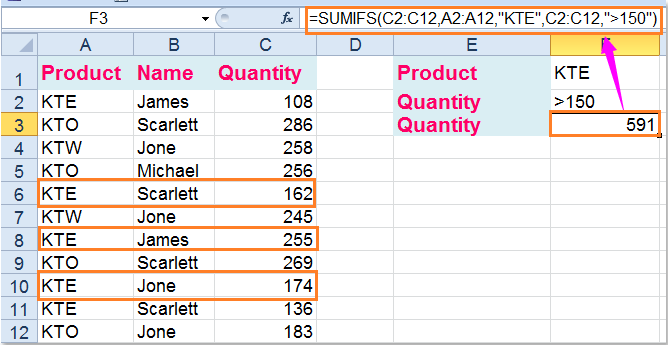You can also us the SUM array formula to get the result you want, =SUM((A2:A12="kte")*(C2:C12>150)*C2:C12) please remember to press Ctrl + Shift + Enter keys after typing this formula.

(4.) Sum the quantity which quantity between 100 and 200

To sum the values between two numbers, please use this formula: =SUMIFS(C2:C12,C2:C12,">100",C2:C12,"<200")( C2:C12 is the range of cells need to sum, C2:C12 , >100 are the first criteria range and criteria, C2:C12, <200, are the second criteria range and criteria), and then press Enter key to get the result as follows: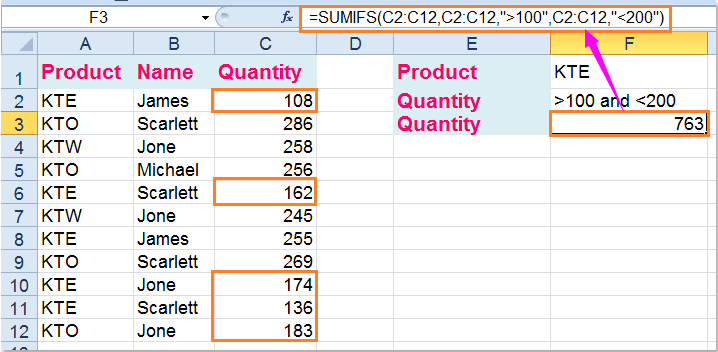Of course, a SUM array formula can help you as well, =SUM((C2:C12>100)*(C2:C12<200)*C2:C12), remember to press Ctrl + Shift + Enter keys together to get the right result.

Related articles:

How to sum multiple columns based on single criteria in Excel?

How to sum cells with text and numbers in Excel?

### Best Office Productivity Tools

#### Supercharge Your Spreadsheets：Experience Efficiency Like Never Before with Kutools for Excel

 Popular Features: Find/Highlight/Identify Duplicates   |  Delete Blank Rows   |  Combine Columns or Cells without Losing Data   |   Round without Formula ... Super Lookup: Multiple Criteria VLookup  |   Multiple Value VLookup  |   VLookup Across Multiple Sheets   |   Fuzzy Lookup .... Advanced Drop-down List: Quickly Create Drop Down List   |  Dependent Drop Down List   |  Multi-select Drop Down List .... Column Manager: Add a Specific Number of Columns   |   Move Columns   |   Unhide Columns   |   Compare Columns to Select Same & Different Cells ... Featured Features: Grid Focus   |  Design View   |   Big Formula Bar   |  Workbook & Sheet Manager   |  Resource Library (Auto Text)   |  Date Picker   |  Combine Worksheets   |  Encrypt/Decrypt Cells   |  Send Emails by List   |  Super Filter   |   Special Filter (filter bold/italic/strikethrough...) ... Top 15 Toolset:  12 Text Tools (Add Text, Remove Characters, ...)   |   50+ Chart Types (Gantt Chart, ...)   |   40+ Practical Formulas (Calculate age based on birthday, ...)   |   19 Insertion Tools (Insert QR Code, Insert Picture from Path, ...)   |   12 Conversion Tools (Numbers to Words, Currency Conversion, ...)   |   7 Merge & Split Tools (Advanced Combine Rows, Split Cells, ...)   |   Many More...

Kutools for Excel boasts over 300 features, ensuring that what you need is just a click away...

Supports Office/Excel 2007-2021 & newer, including 365   |   Available in 44 languages   |   Enjoy a full-featured 30-day free trial.#### Office Tab Brings Tabbed interface to Office, and Make Your Work Much Easier

• Enable tabbed editing and reading in Word, Excel, PowerPoint, Publisher, Access, Visio and Project.
• Open and create multiple documents in new tabs of the same window, rather than in new windows.
• Increases your productivity by 50%, and reduces hundreds of mouse clicks for you every day!